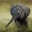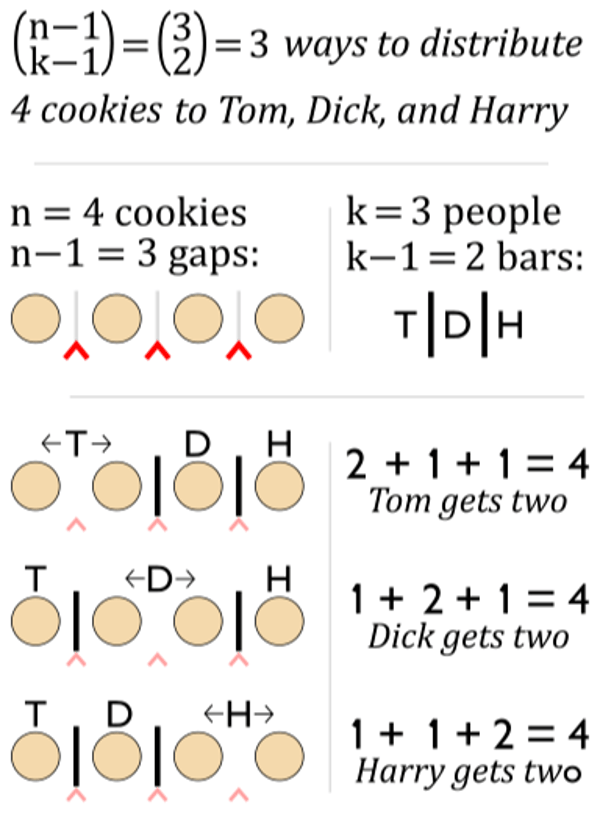Related Tags

c++

# How to find num of positive integral solutions for x1+x2+...+xk=nChayshta Singh

### Overview

In this Answer, we'll learn how to find the number of positive integral solutions for the equation of form x1 + x2 + ... + xk = n.

### Solution

This problem is equivalent to finding the number of ways to distribute $n$ identical objects to $k$ partitions such that each partition has at least one object.

If $ith$ partition contains $xi$ objects, then if we take the sum of sizes of all $k$ partitions, we obtain the identical equation: x1 + x2 + ... + xk = n.

Let's consider a similar example to understand how to solve this combinatorial problem:“Colored circle starsbars 1”, by Guy vandegrift , licensed under CC BY-SA 4.0

We want to distribute 4 cookies to 3 people such that each person gets at least 1 cookie. Each cookie is represented by a colored circle. 4 circles need 3 gaps which are represented by a red caret. Since there are 3 people, we need 2 bars (|) to fill any of the 3 gaps. This problem is now reduced to finding the binomial coefficient:C $\binom{n-1}{k-1} = \frac{(n-1)!}{ (k-1)!( n-k)!}$

In C++ implementation, we use m! = m * (m - 1)! to calculate factorials. We store factorials in unsigned long long type, so we cannot compute values larger than 20!.

### Code example

Let's look at the code below:

#include <iostream>
using namespace std;

//calculates n!, max value of n = 20
unsigned long long factorial(int n){
unsigned long long fact = 1;	//0!= 1, 1!= 1
for(int i = 2; i <= n ; i++){
fact *= i;
}
return fact;
}

int main(){
int k = 3;
int n = 4;
auto ans = (factorial(n - 1)/factorial(k - 1)) / factorial(n-k);
cout << ans; //3
return 0;
}

Code

### Code explanation

• Line 5: The function returns unsigned long long, that is, the maximum integer value it can hold is 9 * 1018. This is less than 21! (= 5 * 1019) and greater than 20!, so we can calculate all values where n < 21.
• Line 8: Infact *= i , the variable fact initially was (i-1)!. After multiplication with i, fact becomes i!.
• Lines 14 and 15 : It defines paramters n and k for equation x1 + x2 + ... + xk = n.
• Line 16: We calculate the binomial coefficient $\binom{n-1}{k-1} = \frac{(n-1)!}{ (k-1)!( n-k)!}$. bina / (b * c) is calculated as (a / b) / c to prevent overflow where     $a = (n -1) ! \hspace{0.15cm}, \hspace{0.15cm} b = (k -1)! \hspace{0.15cm}and \hspace{0.20cm}c = (n - k)!\hspace{0.15cm}.$

### Time complexity

The time complexity of the above problem is $O(n)$ which is the time taken to calculate $n!$.

RELATED TAGS

c++

CONTRIBUTORChayshta Singh
RELATED COURSES

View all Courses

Keep Exploring

Learn in-demand tech skills in half the time